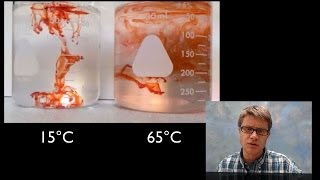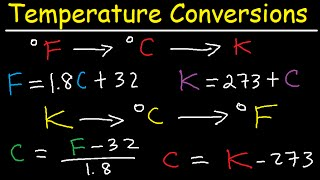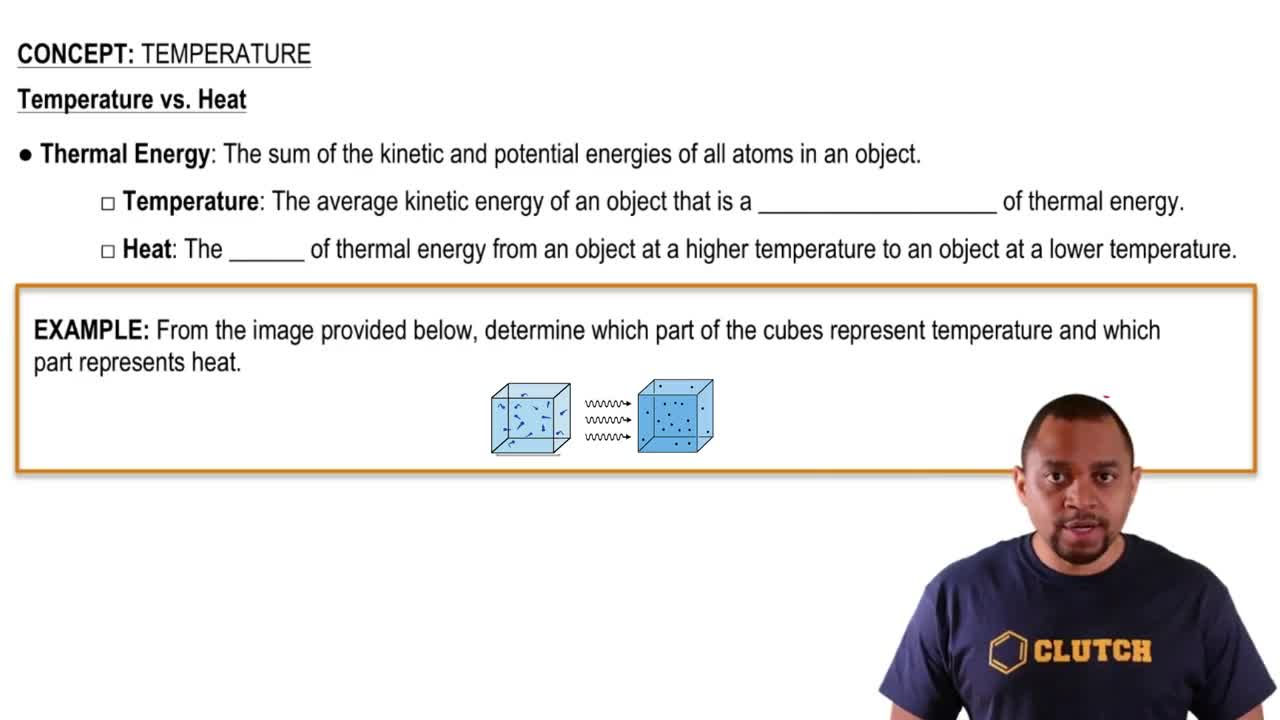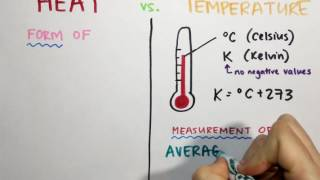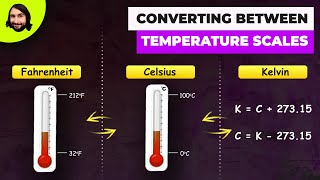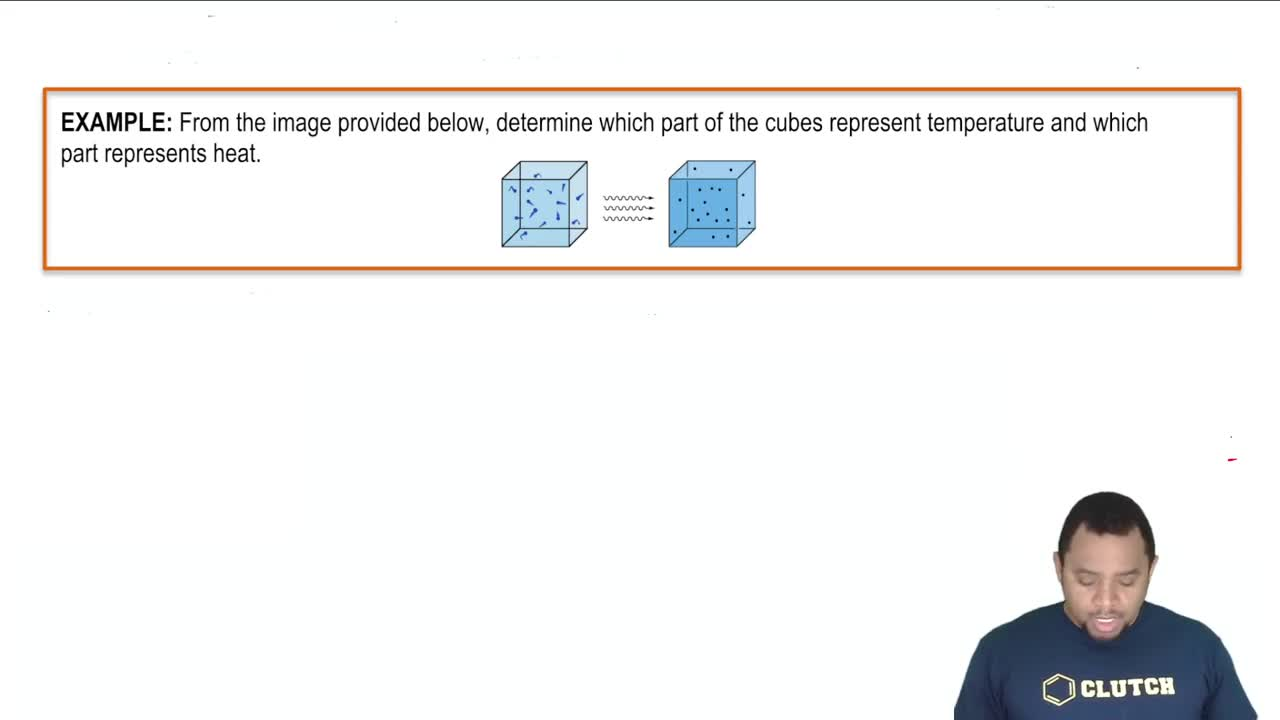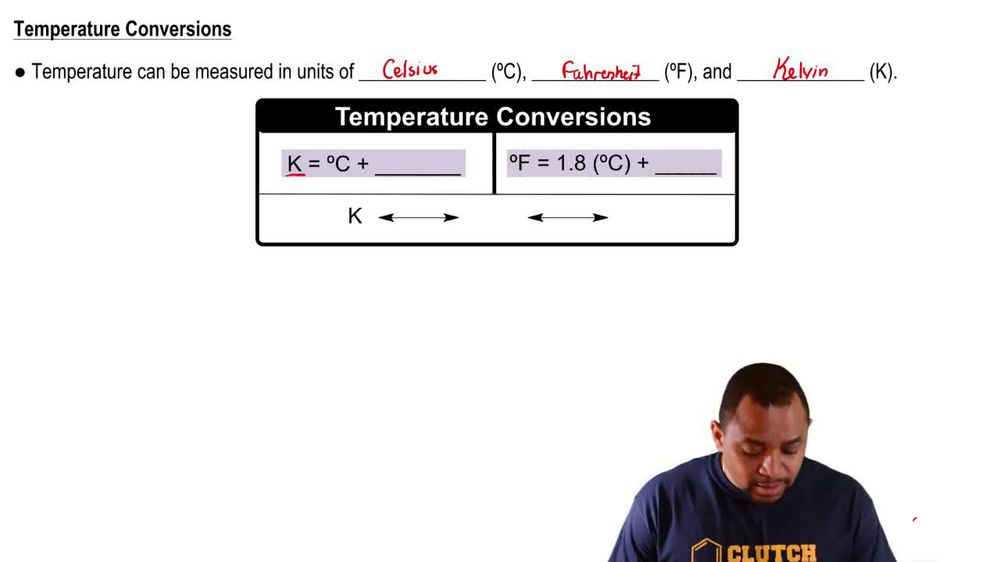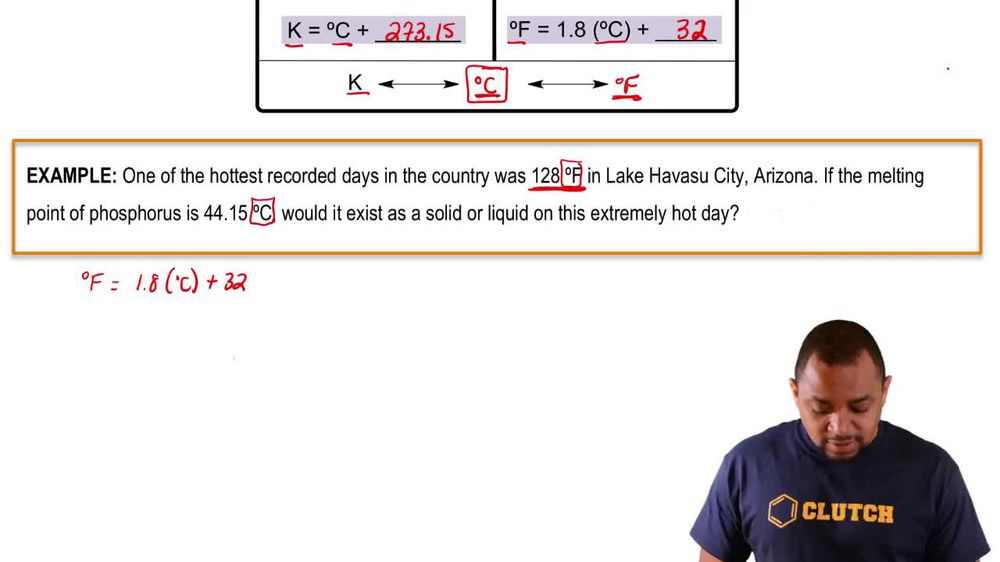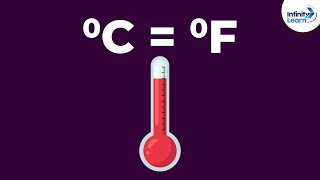Start typing, then use the up and down arrows to select an option from the list.
1. 1. Intro to General Chemistry2. Temperature
Problem

# Sodium chloride has a melting point of 1074 K and a boil-ing point of 1686 K. Convert these temperatures to degrees Celsius and to degrees Fahrenheit.

Relevant Solution1m
Play a video:
Hello everyone for this problem we want to convert 90. Kelvin to degrees Fahrenheit. The equation or formula that we can use to solve this problem is degrees Fahrenheit is equal to kelvin -273. times 9/5 plus 32. So we're given our kelvin here and we just need to plug in and solve. So when we do that, we get our degrees Fahrenheit is equal to 90 0. minus 273. times 9/5 plus 32. Now we can plug this into our calculator and once we do that, we get a final answer of negative 297.3 three degrees Fahrenheit, that is our final answer and that is the end of this problem.